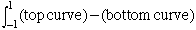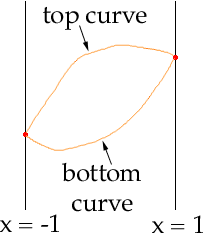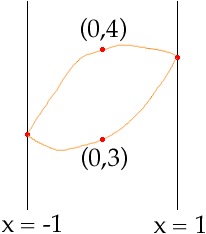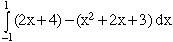hi, phil here. college student studying for degree in IT. need help with maths, especially integration. Thanks question 1 find the area bound by the curves y = x2 + 2x + 3 and y = 2x + 4 question 2 Find the volume generated by rotating the curve x2 + y2 = 9 about the x-axis Hi Phil, For problem like the one you have called question 1 you need to have some rough picture of the graphs of the functions. I want to call the functions f(x) = x2 + 2x + 3 and g(x) = 2x + 4 First find where the two graphs intersect, that is solve x2 + 2x + 3 = 2x + 4. I get x = -1 and x = 1. Thus my interest is the graphs between x = -1 and x = 1 and I know the required area isThe picture in my mind isAll that rremains before actually performing the integration is to determine which is the "top curve" and which is the "bottom curve". To do this I select some x-value between x = -1 and x = 1. In this case the easiest x-value is x = 0. Now find the values of f and g at x = 0. f(0) = 3 and g(0) = 4 Since 4 is larger than 3, g is the top curve and f is the bottom curve.Hence the required area isCheers, Harley Go to Math Central